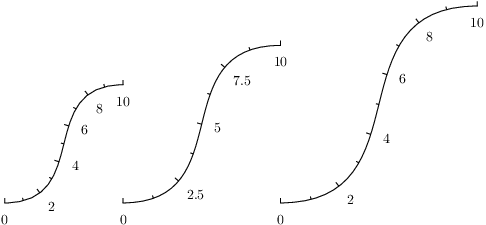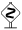# PyX — Example: axis/rating.py

0.4 KB
42.3 KB
35.9 KB
18.2 KB
24.4 KB

## Rater: How a nicely looking axis partition is chosen```from pyx import *

p2 = path.curve(0, 0, 3, 0, 1, 4, 4, 4)
p1 = p2.transformed(trafo.translate(-4, 0).scaled(0.75))
p3 = p2.transformed(trafo.scale(1.25).translated(4, 0))

myaxis = graph.axis.linear(min=0, max=10)

c = canvas.canvas()
c.insert(graph.axis.pathaxis(p1, myaxis))
c.insert(graph.axis.pathaxis(p2, myaxis))
c.insert(graph.axis.pathaxis(p3, myaxis))
c.writeEPSfile("rating")
c.writePDFfile("rating")
c.writeSVGfile("rating")
```

### Description

We here demonstrate how an axis actually chooses its partition.

There are good-looking partitions of an axis and bad-looking ones. The element which chooses one or the other, is the `rater` of an axis. It asks yet another element, the `parter` to suggest several partitions and then chooses the best, according to criteria such as tick distance, number of ticks, subticks, etc. (The partitioning process itself is explained in a later example).

In this example we show the influence of the tick distances: Several axes with the same parameters are plotted on a path which is scaled. Note that the axes choose the ticks appropriately to the available space.

The rating mechanism takes into account the number of ticks and subticks, but also the distances between the labels. Thus, the example in the middle has less ticks than the right version, because there is more space available at the larger path. More interestingly more labels are also shown on the very left path, although it is smaller than the middle one. This is due to the fact, that there is not enough room for labels with a decimal place on the smaller axis!The rating mechanism is configurable and exchangeable by passing rater instances to the `rater` keyword of the axis constructor. But instead of reconfiguring the whole rating mechanism, simple adjustments to favour more or less ticks are easily possible by the axis keyword argument `density`.In this example, the same axis instance is used several times. This works since the axis does not store any data within its own instance. Instead, an `anchoredaxis` instance is created by the `pathaxis` which embeds the axis in a proper environment. This way, a place for storing information for this specific use of the axis instance is provided. When using axes in a graph, the graph instance takes care of the proper setup of the anchored axes instances.